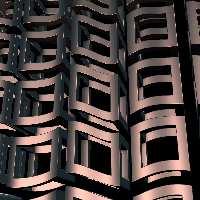# waveWarp

beta
Keywords:
• offset
• shift
• sine
• warp
• wave
filter
waveWarp
RayTK
v0.36

Uses repeating waves to offset space.

The `Axis` parameter determines which axis is used to produce the waves.

A separate wave is used for offsetting for each axis.

The `Period` and `Phase` parameters control the spacing and position of the waves.

The `Amplitude` and `Offset` parameters control how much each axis’s wave shifts coordinates on that axis.

## Parameters

• `Enable`:
• `Wave`:
• `Sine`:
• `Cosine`:
• `Triangle`:
• `Ramp`:
• `Reverse Ramp`:
• `Square`:
• `Additive Square (2)`:
• `Additive Square (4)`:
• `Additive Square (8)`:
• `Wave Axis`: The axis along which the waves are produced.
• `X`:
• `Y`:
• `Z`:
• `Distance From Origin`:
• `Period`: The width of the waves for each axis.
• `Phase`: The phase of the waves for each axis.
• `Amplitude`: The scale of the movement for each axis.
• `Offset`: Offsets the movement for each axis.
• `Phase Offset`:
• `Amplitude Multiplier`:

## Inputs

• `definition_in`: (optional)
• Coordinate Types: `vec2` `vec3`
• Context Types: `Context` `MaterialContext` `CameraContext` `LightContext` `RayContext` `ParticleContext`
• Return Types: `float` `vec4` `Sdf` `Ray` `Light` `Particle`